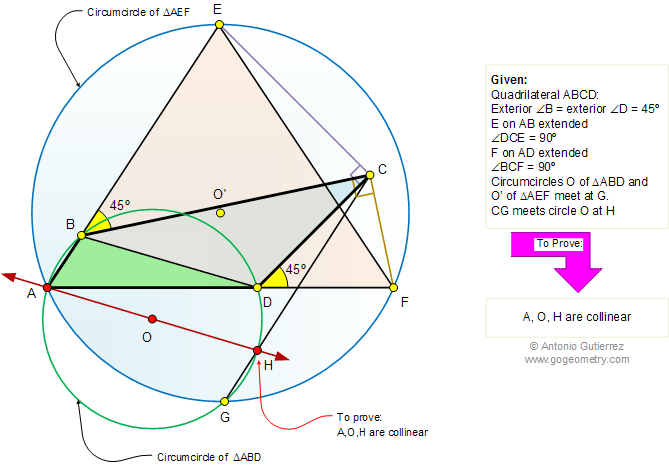Problem 675: Quadrilateral, 45 and 90 Degrees, Circumcircles, Intersecting Circles, Secant, Collinear Points. Level: High School, College, Math Education. The drawing shows the quadrilateral ABCD with the exterior angles B and D equal to 45 degrees. Point E is on AB extended, such that angle DCE = 90 degrees, and point F is on AD extended, such that angle BCF = 90 degrees. CIrcumcircle O of triangle ABD and circumcircle O' of triangle AEF meet at G. CG meets circle O at H. Prove that points A, O, and H are collinear.Geometry problem solving Geometry problem solving is one of the most challenging skills for students to learn. When a problem requires auxiliary construction, the difficulty of the problem increases drastically, perhaps because deciding which construction to make is an ill-structured problem. By “construction,” we mean adding geometric figures (points, lines, planes) to a problem figure that wasn’t mentioned as "given." Recent Additions